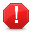# Get or Set Value, Formula, or Formatted Text

Get or Set Value, Formula, or Formatted TextThis article is out of date, please refer to http://books.zkoss.org/wiki/ZK_Spreadsheet_Essentials for more up to date information.

### Purpose

ZK Spreadsheet uses Range.getEditText() to get cell's edit text, and Range.setEditText(String)to set cell's text as input by the user.

### ZUML

```<zk>
<div width="100%" height="100%" apply="demo.EditorComposer" >
<div height="3px"></div>
<combobox id="focusCombobox" mold="rounded"	style="text-align:center;">
</combobox>
<textbox id="formulaEditor" cols="100"/>
<div height="3px"></div>
maxrows="200"
maxcolumns="40"
width="100%"
</div>
</zk>
```

### Composer

#### Current Cell Text

We can use onCellFocused event to get the current focus cell and display the cell's text.

```public void onCellFocused\$spreadsheet(CellEvent event) {
int row = event.getRow();
int col = event.getColumn();

currentCell = Utils.getCell(sheet, row, col);
currentRange = Ranges.range(sheet, row, col);
formulaEditor.setText(currentRange.getEditText());
}
```

#### Cell Position

First, we need to get the current cell's position. We can get row and column index from CellEvent, then use Spreadsheet.getRowtitle(String) and Spreadsheet.getColumntitle((String) to get the current cell's title.

```public void onCellFocused\$spreadsheet(CellEvent event) {
int row = event.getRow();
int col = event.getColumn();
...
```

#### Cell Value

After we get the cell's position, we can get the cell's value from Range.getEditText()

```public void onCellFocused\$spreadsheet(CellEvent event) {
...

currentCell = Utils.getCell(sheet, row, col);
currentRange = Ranges.range(sheet, row, col);
formulaEditor.setText(currentRange.getEditText());
}
```

#### Editor

For editor, we can use the onOK event, which menus when the user click Enter keyboard, set cell's value and make spreadsheet's focus to the next cell

```public void onOK\$formulaEditor() {
currentRange.setEditText(formulaEditor.getText());
}
```

Second, we can also use the onChange and onChanging event to get the current editor's value and set the cell's value.

```public void onChanging\$formulaEditor(InputEvent event) {
if (currentCell.getCellType() != Cell.CELL_TYPE_FORMULA)
currentRange.setEditText(event.getValue());
}

public void onChange\$formulaEditor() {
currentRange.setEditText(formulaEditor.getText());
}
```

View the complete source of ZUML editor.zul

View the complete source of composer EditorComposer.java

# Version History

Last Update : 2022/01/19

Version Date Content

All source code listed in this book is at Github.